# Uniform Circular Motion

#### Uniform Circular Motion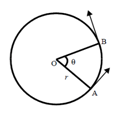When a particle moves in a circle with a constant speed then the motion is known as uniform circular motion.

We can consider an object moving in a circle with a uniform speed a round a fixed point O as centre. If the object moves from A to B so that the radius OA moves through an angle θ, its angular velocity (ω) about O is defined as the rate at which the radius vector sweeps. If t is the time taken by the object to move from A to B then,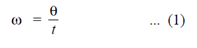The unit of the angular velocity is rad s-1. The time taken T by the object to describe the circle once is called the period of circular motion. It is given by: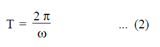If s is the length of the arc AB, then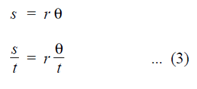The linear velocity v of the rotating object is given by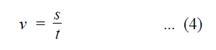Substituting equation (1) and (4) in (3), we get:This is the relation connecting the linear velocity and the angular velocity of the object in circular motion.

#### Centripetal Force

• Centripetal force is the force that is directed toward the center of an orbital path/spinning object which keeps the revolving object in its orbit. This is in opposition to the “centrifugal force” – a kind of fictitious force that appears to try to pull the object away from the center of the orbit (due to inertia).
• The centripetal force causes acceleration towards the centre of the circle and this acceleration is called the centripetal acceleration.
• An artificial satellite orbiting around the earth does not fall down. This is so because the attraction of earth provides the necessary acceleration for its motion.
• Both Centripetal Force and Centrifugal Force play role in a Washing Machine
• Wheel of an automobile spins in mud because the centripetal force is not enough to hold the mud on tyre.

When we swing a stone tied to a string, we observe that the stone follows a circular path. But when we let the string slip through our fingers, we observe that the stone no longer follows a circular path but flies off in the direction of the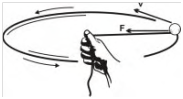instantaneous velocity. This direction is tangential to the circular path. We conclude that a force acting on the stone pulls it towards the centre of the circle. This force is called the centripetal force. Centripetal force is the force needed to make an object travel in the circular path. The centripetal force causes acceleration towards the centre of the circle and this acceleration is called the centripetal acceleration. The centripetal acceleration (ac) of an object in uniform circular motion is given byIf m is the mass of the object then the centripetal force (F) is given by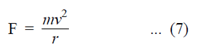In terms of angular velocity (w) of the object, equation (7) becomes#### Examples of Centripetal Force

We suppose that we have a string which can be broken if we exert a force of 1500 Newton. If we attach an object with mass 8.3 kilogram at one of its end and whirl it horizontal direction in a circle of 80 cm, then what should be the maximum speed it can have, so that the string does not break.

The above equation will be arranged as follows:The above description was taught to us in our school days to explain why bucket carrying water is, when rotated in a vertical circle does not fall downward when the bucket is at highest point. This is because the necessary “centripetal” force is provided by the motion. When moon revolves around the earth, centripetal force is provided by gravitational force of Earth on moon. Similar is with artificial satellites, because as they move around the earth, the force of attraction of earth on the satellite provides necessary centripetal force.

#### Practical Applications of Centripetal Force

##### Artificial Satellites

Gravitational force between a satellite and the earth acts as a centripetal force, keeping the satellite in orbit

##### Washing Machines – Spin Dryer

The spin dryer of a washing machine removes excess water from the clothing by rotating at a high speed. The high speed of rotation creates a high centrifugal force for the water in the clothing which causes it to be pulled to the outside of the spinning portion of the washing machine and away from the clothes. However, it is the Centripetal force that keeps the clothes themselves away from the outer portion of the washing machine. This is provided by the walls of the rotating spin dryer. Since there is insufficient centripetal force affecting the water (only friction & surface tension holding it to the clothes), it flows to the outer walls and is separated from the clothes, which removes the excess water.

The less desirable case of lack of centripetal force is when the rear wheel of an automobile spins in mud. The adhesion of the mud to the wheel which is the centripetal force in this case is not enough to hold the mud on the tyre. So it comes off tangentially to the tyre’s circular motion.

#### Centrifugal force

• Centrifugal force is in opposite direction to Centripetal force. On earth, it is minimum at poles and maximum at equator.
• In centrifuges, heavier particles move away from the centre while lighter particles remain near axis of rotation.
• When a sample of blood is centrifuged, the red blood cells accumulate at the bottom, because red blood cells are heavier than White Blood Cells.

We feel a force pushing us outward or away from the centre of curvature when we travel in a fast moving car rounding a sharp curve or in a rotating ride in an amusement park. This force is known as centrifugal force. It acts in the opposite direction to that of centripetal force. The centrifugal force is also given by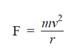When earth rotates about its own axis, the velocity of bodies near the equator is more than that at the poles. On earth the centrifugal force is minimum at the poles and maximum at the equator. This is the reason why the poles of the earth are found to be nearly flat and the diameters of earth along the equator and poles are different by 48 km. The centripetal force required for a car or a bicycle to go round a circular curve depends on its speed and the radius of curvature of the curve.

#### Applications of Centrifugal Force

##### Centrifuges

The principle of centrifugal force is applied to the machines called centrifuges. They are used to separate materials of different weights or densities by spinning action. The liquid is rotated in a cylindrical vessel at a high speed with the help of an electric motor. The heavier particles move away from the axis of rotation and lighter particles remain nearer to the axis of rotation

##### Washing Machines

The spinning drum in a washing machine to separate water from clothes is a centrifuge

##### Other applications

Centrifuges are used in separating blood cells from plasma. When blood samples are centrifuged, the heavier red cells reach the bottom and lighter white cells go to the top of the tube.

Cream from milk is separated by centrifuges in dairy separators. Ultra centrifuges with speeds of the order of 5 x 105 rpm are used to concentrate viruses in solution. Centrifuges are used in Uranium enrichment. Sugar crystals are separated from molasses with the help of a centrifuge. Honey is also separated from bees wax with the help of a centrifuge.

If a vehicle moves at very high speed over a curved path, the centrifugal force makes it topple. This is because the centrifugal force overcomes the frictional force between the road and the tyres of the vehicle. To prevent this, the curved tracks are always banked. It means that the outer edge of the road is slightly elevated at an angle Θ. This angle of elevation is given by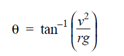Where g is acceleration due to gravity. Due to banking of curves the centrifugal force balances with frictional force and equilibrium is reached. Thus toppling of vehicles is prevented on curved roads. This is known as banking of tracks. The racing track is designed like a concave disc for the same reason.

In circus there during the cage of death event, a motor cyclist drives a motor cycle at a high speed on the inner walls of a spherical cage of iron. But he does not fall off the motor cycle even when he is upside down. The centrifugal force keeps the motor cyclist glued to his seat while driving his motor cycle inside the cage.

••